xMagnetic dipoleEncyclopedia
A magnetic dipole is the limit of either a closed loop of electric current
Electric current
Electric current is a flow of electric charge through a medium.This charge is typically carried by moving electrons in a conductor such as wire...

or a pair of poles as the dimensions of the source are reduced to zero while keeping the magnetic moment
Magnetic moment
The magnetic moment of a magnet is a quantity that determines the force that the magnet can exert on electric currents and the torque that a magnetic field will exert on it...

constant. It is a magnetic analogue of the electric dipole
Electric dipole moment
In physics, the electric dipole moment is a measure of the separation of positive and negative electrical charges in a system of charges, that is, a measure of the charge system's overall polarity with SI units of Coulomb-meter...

, but the analogy is not complete. In particular, a magnetic monopole
Magnetic monopole
A magnetic monopole is a hypothetical particle in particle physics that is a magnet with only one magnetic pole . In more technical terms, a magnetic monopole would have a net "magnetic charge". Modern interest in the concept stems from particle theories, notably the grand unified and superstring...

, the magnetic analogue of an electric charge
Electric charge
Electric charge is a physical property of matter that causes it to experience a force when near other electrically charged matter. Electric charge comes in two types, called positive and negative. Two positively charged substances, or objects, experience a mutual repulsive force, as do two...

, has never been observed. Moreover, one form of magnetic dipole moment is associated with a fundamental quantum property, the spin
Spin (physics)
In quantum mechanics and particle physics, spin is a fundamental characteristic property of elementary particles, composite particles , and atomic nuclei.It is worth noting that the intrinsic property of subatomic particles called spin and discussed in this article, is related in some small ways,...

of elementary particles.

The magnetic field
Magnetic field
A magnetic field is a mathematical description of the magnetic influence of electric currents and magnetic materials. The magnetic field at any given point is specified by both a direction and a magnitude ; as such it is a vector field.Technically, a magnetic field is a pseudo vector;...

around any magnetic source looks increasingly like the field of a magnetic dipole as the distance from the source increases.

## External magnetic field produced by a magnetic dipole moment

In classical physics
Classical physics
What "classical physics" refers to depends on the context. When discussing special relativity, it refers to the Newtonian physics which preceded relativity, i.e. the branches of physics based on principles developed before the rise of relativity and quantum mechanics...

, the magnetic field of a dipole is calculated as the limit of either a current loop or a pair of charges as the source shrinks to a point while keeping the magnetic moment constant. For the current loop, this limit is most easily derived for the vector potential. Outside of the source region, this potential is (in SI units)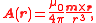and the magnetic flux density (strength of the B-field) in teslas is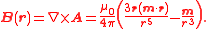Alternatively one can obtain the scalar potential first from the magnetic pole limit,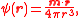and hence the magnetic field strength (or strength of the H-field) in ampere-turns
Ampere-turn
The ampere-turn was the MKS unit of magnetomotive force , represented by a direct current of one ampere flowing in a single-turn loop in a vacuum...

per meter is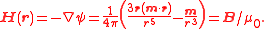The magnetic field is symmetric under rotations about the axis of the magnetic moment.

## Internal magnetic field of a dipole

The two models for a dipole (current loop and magnetic poles) give the same predictions for the magnetic field far from the source. However, inside the source region they give different predictions. The magnetic field between poles is in the opposite direction to the magnetic moment (which points from the negative charge to the positive charge), while inside a current loop it is in the same direction (see the figure to the right). Clearly, the limits of these fields must also be different as the sources shrink to zero size. This distinction only matters if the dipole limit is used to calculate fields inside a magnetic material.

If a magnetic dipole is formed by making a current loop smaller and smaller, but keeping the product of current and area constant, the limiting field is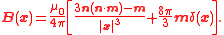Unlike the expressions in the previous section, this limit is correct for the internal field of the dipole.

If a magnetic dipole is formed by taking a "north pole" and a "south pole", bringing them closer and closer together but keeping the product of magnetic pole-charge and distance constant, the limiting field is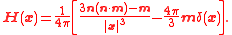These fields are related by , where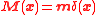is the magnetization
Magnetization
In classical electromagnetism, magnetization or magnetic polarization is the vector field that expresses the density of permanent or induced magnetic dipole moments in a magnetic material...

.

## Forces between two magnetic dipoles

The force exerted by one dipole moment on another separated in space by a vector can be calculated using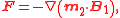or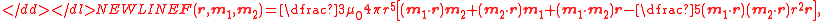where is the distance between dipoles. The force acting on is in the opposite direction.

The torque can be obtained from the formula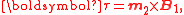which gives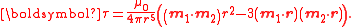## Dipolar fields from finite sources

The magnetic scalar potential
Magnetic potential
The term magnetic potential can be used for either of two quantities in classical electromagnetism: the magnetic vector potential, A, and the magnetic scalar potential, ψ...

produced by a finite source, but external to it, can be represented by a multipole expansion
Multipole expansion
A multipole expansion is a mathematical series representing a function that depends on angles — usually the two angles on a sphere. These series are useful because they can often be truncated, meaning that only the first few terms need to be retained for a good approximation to the original...

. Each term in the expansion is associated with a characteristic moment and a potential having a characteristic rate of decrease with distance from the source. Monopole moments have a rate of decrease, dipole moments have a rate, quadrupole moments have a rate, and so on. The higher the order, the faster the potential drops off. Since the lowest-order term observed in magnetic sources is the dipolar term, it dominates at large distances. Therefore, at large distances any magnetic source looks like a dipole with the same magnetic moment
Magnetic moment
The magnetic moment of a magnet is a quantity that determines the force that the magnet can exert on electric currents and the torque that a magnetic field will exert on it...

.